# The Identity Property of Addition

The property is pretty simple – but let’s explore why it matters!Author
Taylor HartleyExpert Reviewer

Published: August 24, 2023# The Identity Property of Addition

The property is pretty simple – but let’s explore why it matters!Author
Taylor HartleyExpert Reviewer

Published: August 24, 2023# The Identity Property of Addition

The property is pretty simple – but let’s explore why it matters!Author
Taylor HartleyExpert Reviewer

Published: August 24, 2023Key takeaways

• Adding zero to anything is simple, but foundational – Whether you’re dealing with complex negative numbers and fractions or simple whole numbers, adding zero is the same across the board.
• There are different ways to ask for the identity property of addition – You may run across the term “additive identity property,” too.
• Memorization is key – Once you know that adding zero to any number results in the number itself, you’ll be solving these simple equations in a snap!

The identity property of addition is one of the most basic concepts in math. Without this skill, it will be almost impossible to move on to more complicated math concepts like adding multiple whole numbers, subtracting, multiplying, and dividing.

It’s very simple — when you add zero to any number, the result is always the number itself. Let’s dig in to learn more about the identity property of addition.

## What is the identity property of addition?The identity property of addition states that by adding 0 to any number, the result is the number itself. In other words, when we add 0 to any number, it does not change the number, therefore keeping its identity. The identity property of addition is sometimes also referred to as the additive identity property.

## The additive identity property formulaYou already know that addition is the process of adding two or more numbers together, so this concept should be a breeze! With the additive identity property, zero is known as the identity element in this property. This property can be applied to real numbers, complex numbers, integers, rational numbers, and more.

To give you a visual, if P is any real number, then we can express the additive identity property as the following:

P + 0 = P = 0 + P

Looking for a simpler formula? The identity property of addition can be written as a + 0 = a. Here, you can see that when any number is added to zero, the sum is simply the number itself. For example, if we add 0 to 3, we get 3 as the sum.

3 + 0 = 3.

### The additive identity property with whole numbersLet’s walk through what happens with whole numbers. Again here, the additive identity of whole numbers is zero. When zero is added to a whole number, the sum is the number itself. To check this property with a whole number, let’s use 38 as an example.

38 + 0 = 38

### The additive identity property with negative numbersThe additive identity of negative numbers is simple — don’t let the negative sign fool you! If a zero is added to any negative number, the sum is the negative number. For example, if we add zero to -54, the sum is -54.

0 + -54 = -54

### The additive identity property with fractionsFinally, let’s tackle fractions. Again, don’t let fractions scare you! The additive identity of fractions is, you guessed it, zero. If you add zero to any fraction, the sum is the fraction. For example, if we add zero to ¼, the sum is ¼.

0 + ¼  =  ¼

## Let’s practice togetherThe best way to learn about the identity property of addition is to practice! Below, let’s take a look at a few practice problems.

### 1. Fill in the missing number: 358 + ___ = 358Using the additive identity property, we can fill in the missing number 0.

### 2. Fill in the missing number: 0 + ___ = ⅝We know that the additive identity property for negative numbers and fractions is 0. Therefore, we can fill in the missing number which is ⅝.

### 3. True or false? The additive identity property is also known as the identity property of addition.True. The additive identity property is also known as the identity property of addition.

### 4. What is the sum of 0 + -3?The sum is -3 because we know that the sum of zero and any negative number is simply the negative number.

Ready to give it a go?

Now that we know what the identity property of addition is and read several examples together, you’re ready to do a few more practice problems to put your new skills to the test! Work through the following problems on your own. Feel free to look back at the practice problems above if you get stuck, or if you need a quick reminder on the additive identity property.

## Practice problems

The answer is a + (0) = a.

## Parent Guide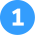How did we get here?

1. Recognize that the additive identity of whole numbers is zero.
2. Find the whole number to find the sum which is 1,398.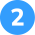How dd we get here?

1. Recognize that the additive identity of negative numbers and fractions is zero.
2. Find the integer to find the missing number which is – ¾ .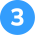How did we get here?

1. Recognize the numbers in the equation. One is a whole number and one is zero.
2. Remember that the additive identity of whole numbers is zero.
3. Solve the equation. 78 + 0 = 78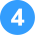How did we get here?

1. Recognize that the additive identity of whole numbers is zero.
2. Find the whole number in the equation and in the sum. This means that you’d have to add 0 for the result to be the same whole number.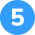The answer is a + (0) = a

How did we get here?

1. You know that when any number is added to zero, the sum is simply the number itself.
2. Simply substitute a letter for a number to write a formula.

## FAQs about math strategies for kidsWe understand that diving into new information can sometimes be overwhelming, and questions often arise. That’s why we’ve meticulously crafted these FAQs, based on real questions from students and parents. We’ve got you covered!

The identity property of addition states that when you add zero to any number, the sum is equal to the original number. So, take the number 5. If we add zero to 5, the sum will still be 5. So, 5 + 0 = 5.

Similarly, if we take any other number, such as 10, and add zero to it, the result will remain the same. So, 10 + 0 = 10.

The commutative property of addition states that the order in which numbers are added does not affect the result. In other words, when you add two numbers, you can change the order of the numbers in the equation without changing the sum.## Related Posts

Title 1

Title 2

Title 3

Lesson credits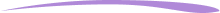Taylor Hartley

Taylor Hartley is an author and an English teacher based in Charlotte, North Carolina. When she's not writing, you can find her on the rowing machine or lost in a good novel.

Jill Padfield has 7 years of experience teaching high school mathematics, ranging from Alegra 1 to AP Calculas. She is currently working as a Business Analyst, working to improve services for Veterans while earning a masters degree in business administration.

Taylor Hartley

Taylor Hartley is an author and an English teacher based in Charlotte, North Carolina. When she's not writing, you can find her on the rowing machine or lost in a good novel.

Jill Padfield has 7 years of experience teaching high school mathematics, ranging from Alegra 1 to AP Calculas. She is currently working as a Business Analyst, working to improve services for Veterans while earning a masters degree in business administration.# Are you a parent, teacher or student?

Are you a parent or teacher?

## Hi there!

Book a chat with our team